# The length

The length of the circle is 24 cm. What is the length of the circular arc of the corresponding angle 30°?

x =  2 cm

### Step-by-step explanation: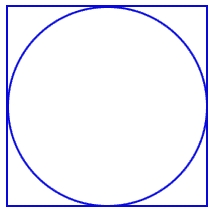Did you find an error or inaccuracy? Feel free to write us. Thank you!Tips to related online calculators
Need help to calculate sum, simplify or multiply fractions? Try our fraction calculator.

#### You need to know the following knowledge to solve this word math problem:

We encourage you to watch this tutorial video on this math problem:

## Related math problems and questions:

• Circular arcCalculate the length of the circular arc if the diameter d = 20cm and the angle alpha = 142 °
• ArcCircle arc corresponding to angle is 32° is 28 dm long. What is the length of the entire circle?
• Central angleWhat is the length of the arc of a circle with a diameter of 46 cm, which belongs to a central angle of 30°?
• Length of the arcWhat is the length of the arc of a circle k (S, r=68mm), which belongs to a central angle of 78°?
• The central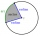The central angle of a sector is 30° and the radius is 15 m. Determine its perimeter.
• Minute handWhat is the distance the minute hand of the clock travels in 12 minutes, if the diameter of the clock is 30 cm and the hand extends to a distance of 2 cm from the edge of the clock?
• TrianglesFind out whether given sizes of the angles can be interior angles of a triangle: a) 23°10',84°30',72°20' b) 90°,41°33',48°37' c) 14°51',90°,75°49' d) 58°58',59°59',60°3'
• Disc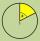The circumference of the disk is 78.5 cm. What is the circumference of the circular arc of 32° on the disc?
• Clocks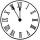What distance will describe the tip of minute hand 6 cm long for 20 minutes when we know the starting position with finally enclose hands each other 120°?
• Quarter circularThe wire that is hooked around the perimeter of quarter-circular arc has length 3π+12. Determine the radius of circle arc.
• Rectangular trapeziumCalculate the perimeter of a rectangular trapezium when its content area is 576 cm2 and sice a (base) is 30 cm, height 24 cm.
• Circular segmentCalculate the area S of the circular segment and the length of the circular arc l. The height of the circular segment is 2 cm and the angle α = 60°. Help formula: S = 1/2 r2. (Β-sinβ)
• Track arc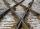Two straight tracks is in an angle 74°. They will join with circular arc with radius r=1127 m. How long will be arc connecting these lines (L)? How far is the center point of arc from track crossings (x)?
• Irrigation sprinklerThe irrigation sprinkler can twist with an angle of 320° and has a reach of 12 meters. Which area can irrigate?
• Arc and segmentCalculate the length of circular arc l, area of the circular arc S1 and area of circular segment S2. Radius of the circle is 11 and corresponding angle is (2)/(12) π.
• Arc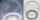The length of the circle is 41 amd arc length of the circle 9. What is the magnitude of the angle of this arc?
• Arc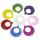What area of a circle occupied the flowers planted in the arc of a circle with radius 3 m with central angle 45°?